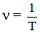Ex﻿

# 5. Sound

#### Relation Between Wave Velocity, Frequency, and Wave Length for a Periodic Waveor...(1)
sinceequation (1) can also be written as
v = ν λ ...(2)
Wave Velocity = Frequency × Wave Length

Example : 1
If 50 waves are produced in 2 seconds, what is its frequency ?
Solution. Frequency,= 50/2 = 25 Hz
Example : 2
A source produce 50 crests and 50 troughs in 0.5 second. Find the frequency.
Solution. 1 crest and 1 trough = 1 wave
∴ 50 crests and 50 troughs = 50 waves
Now, Frequency,If you want to give information about online courses to other students, then share it with more and more on Facebook, Twitter, Google Plus. The more the shares will be, the more students will benefit. The share buttons are given below for your convenience.
×

#### NTSE Physics (Class X)

• Electricity
• Magnetic Effect of Curent
• Light Reflection
• Light Refraction
• Human Eye & Colourful World
• Source of Energy
• Motion

#### NTSE Physics (Class IX)

• Force & laws of motion
• Work and Energy
• Gravitation
• Sound

SHOW CHAPTERS